# 5. Plotting Simple Features

For a better version of the sf vignettes see https://r-spatial.github.io/sf/articles/

This vignette describes the functions in `sf` that can help to plot simple features. It tries to be complete about the plot methods `sf` provides, and give examples and pointers to options to plot simple feature objects with other packages (mapview, tmap, ggplot2).

# Plot methods for `sf` and `sfc` objects

## Geometry only: `sfc`

Geometry list-columns (objects of class `sfc`, obtained by the `st_geometry` method) only show the geometry:

``````library(sf)
demo(nc, ask = FALSE, echo = FALSE)``````
``````## Reading layer `nc.gpkg' from data source `/tmp/Rtmpl6EaFo/Rinst6231414d3e82f/sf/gpkg/nc.gpkg' using driver `GPKG'
## Simple feature collection with 100 features and 14 fields
## Attribute-geometry relationship: 0 constant, 8 aggregate, 6 identity
## Geometry type: MULTIPOLYGON
## Dimension:     XY
## Bounding box:  xmin: -84.32385 ymin: 33.88199 xmax: -75.45698 ymax: 36.58965
## Geodetic CRS:  NAD27``````
``plot(st_geometry(nc))``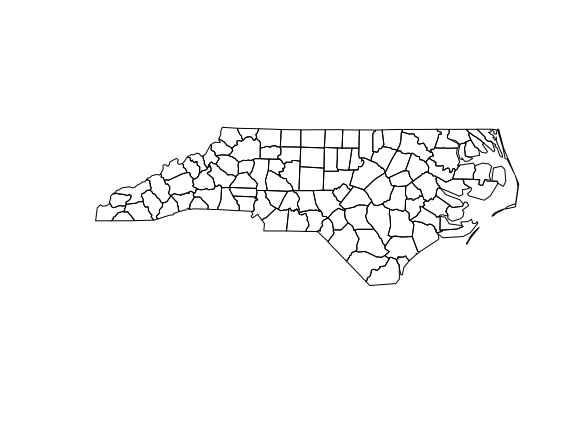which can be further annotated with colors, symbols, etc., as the usual base plots, e.g. points are added to a polygon plot by:

``````plot(st_geometry(nc), col = sf.colors(12, categorical = TRUE), border = 'grey',
axes = TRUE)
plot(st_geometry(st_centroid(nc)), pch = 3, col = 'red', add = TRUE)``````
``````## Warning in st_centroid.sf(nc): st_centroid assumes attributes are constant over
## geometries of x``````
``````## Warning in st_centroid.sfc(st_geometry(x), of_largest_polygon =
## of_largest_polygon): st_centroid does not give correct centroids for longitude/
## latitude data``````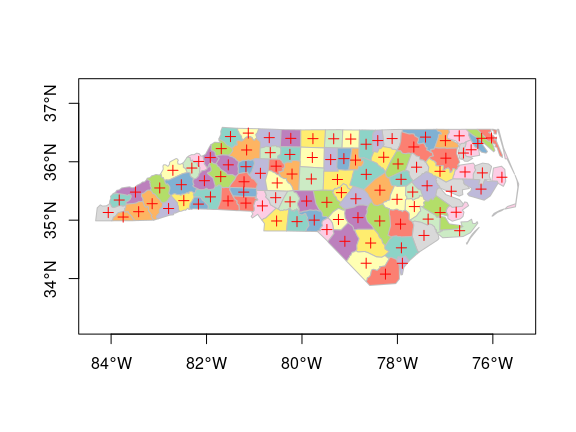and legends, titles and so on can be added afterwards. `border=NA` removes the polygon borders.

As can be seen, the axes plotted are sensitive to the CRS, and in case of longitude/latitude coordinates, degree symbols and orientation are added if `axes = TRUE`.

## Geometry with attributes: `sf`

The default plot of an `sf` object is a multi-plot of all attributes, up to a reasonable maximum:

``plot(nc)``
``````## Warning: plotting the first 10 out of 14 attributes; use max.plot = 14 to plot
## all``````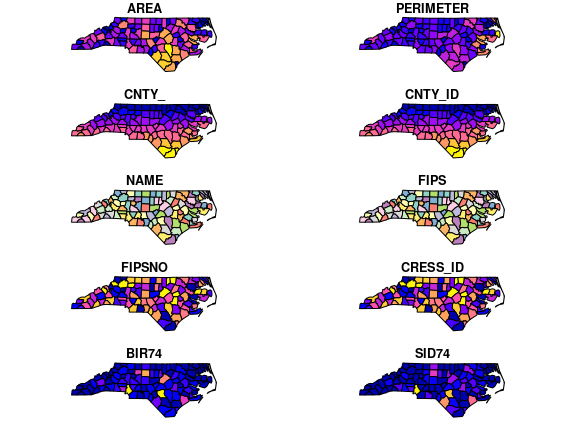with a warning when not all attributes can be reasonably plotted. One can increase the maximum number of maps to be plotted by

``plot(nc, max.plot = 14)``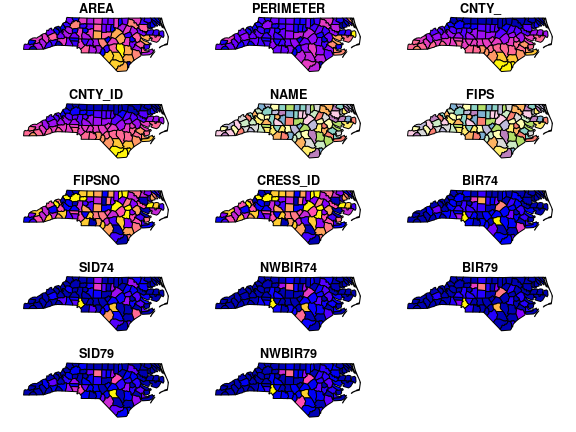The row/column layout is chosen such that the plotting area is maximally filled. The default value for `max.plot` can be controlled, e.g. by setting the global option `sf_max.plot`:

``````options(sf_max.plot=1)
plot(nc)``````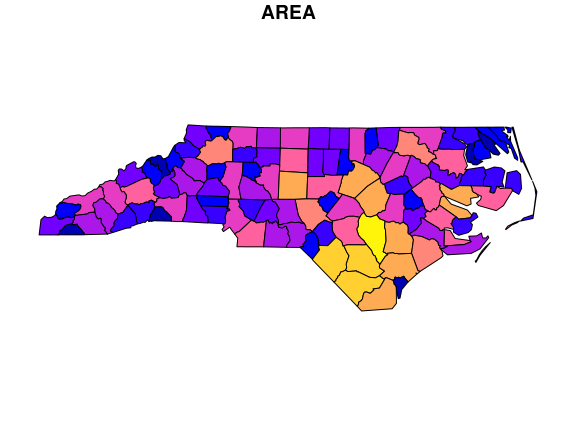# Color key place and size

In case a single attribute is selected, by default a color key is given the side of the plot where it leaves as much as possible room for the plotted map; for `nc` this is below:

``plot(nc["AREA"])``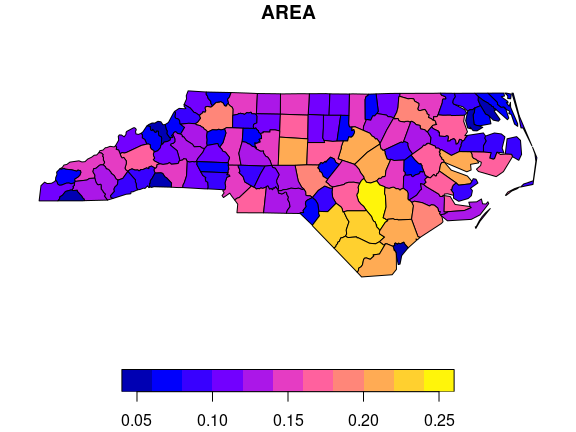but this can be controlled, and set to a particular side (1=below, 2=left, 3=above and 4=right):

``plot(nc["AREA"], key.pos = 4)``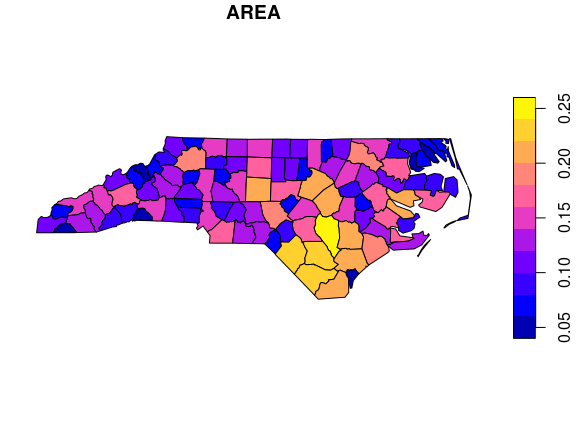The size of a color key can be controlled, using either relative units (a number between 0 and 1) or absolute units (like `lcm(2)` for 2 cm):

``plot(nc["AREA"], key.pos = 1, axes = TRUE, key.width = lcm(1.3), key.length = 1.0)``